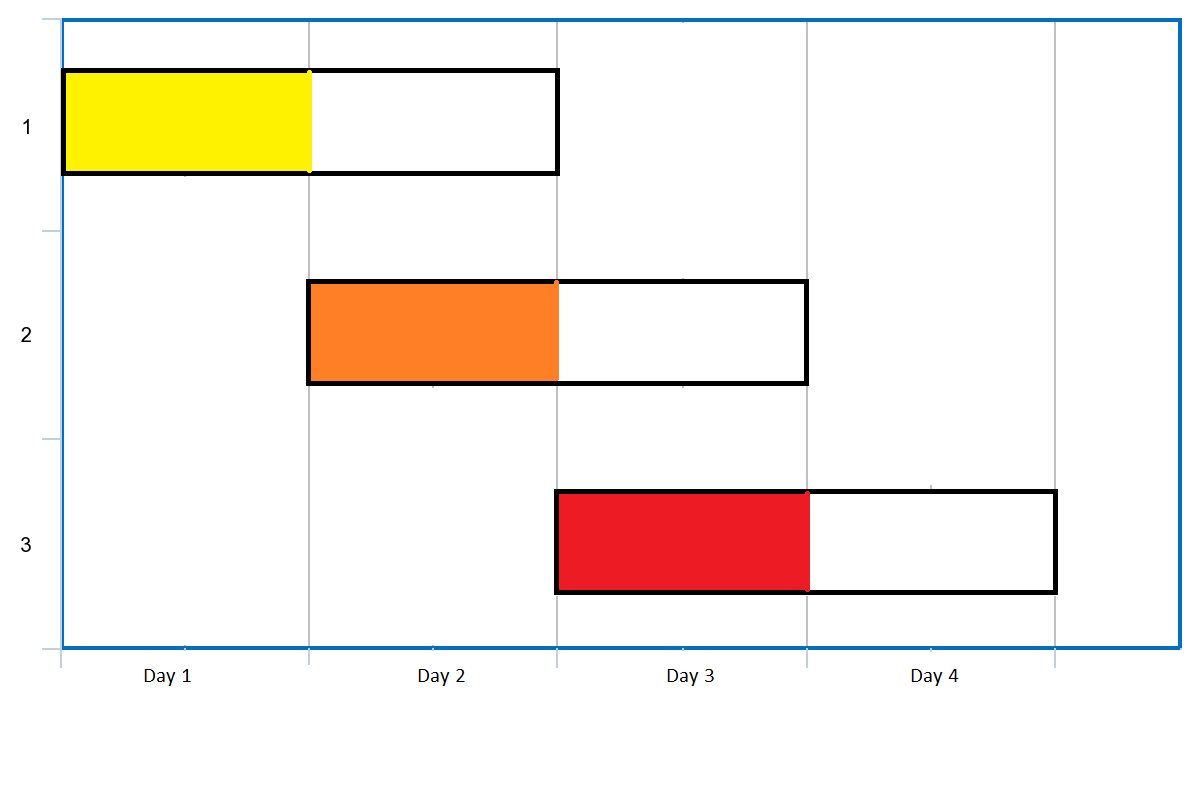# GeetCode Hub

Given an array of `events` where `events[i] = [startDayi, endDayi]`. Every event `i` starts at `startDayi` and ends at `endDayi`.

You can attend an event `i` at any day `d` where `startTimei <= d <= endTimei`. Notice that you can only attend one event at any time `d`.

Return the maximum number of events you can attend.

Example 1:```Input: events = [[1,2],[2,3],[3,4]]
Output: 3
Explanation: You can attend all the three events.
One way to attend them all is as shown.
Attend the first event on day 1.
Attend the second event on day 2.
Attend the third event on day 3.
```

Example 2:

```Input: events= [[1,2],[2,3],[3,4],[1,2]]
Output: 4
```

Example 3:

```Input: events = [[1,4],[4,4],[2,2],[3,4],[1,1]]
Output: 4
```

Example 4:

```Input: events = [[1,100000]]
Output: 1
```

Example 5:

```Input: events = [[1,1],[1,2],[1,3],[1,4],[1,5],[1,6],[1,7]]
Output: 7
```

Constraints:

• `1 <= events.length <= 105`
• `events[i].length == 2`
• `1 <= startDayi <= endDayi <= 105`

class Solution { public int maxEvents(int[][] events) { } }